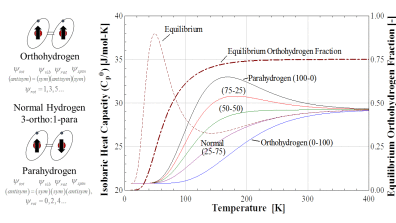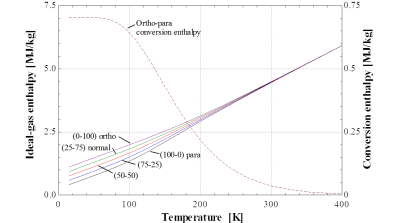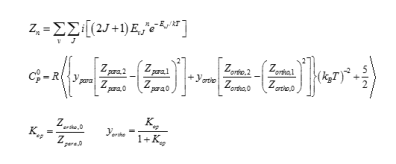As you already know, hydrogen is unique among fluids for a number of reasons. I like to introduce one of these key differences from the historical perspective:

In 1932, Werner Heisenberg won the Nobel Prize in physics “for the creation of quantum mechanics, the application of which has, inter alia, led to the discovery of the allotropic forms of hydrogen.” These allotropic forms of hydrogen called orthohydrogen and parahydrogen exist due to parity between the nuclear spin and rotational spin function for the hydrogen molecule. Orthohydrogen is a higher energy triplet restricted to odd rotational energy levels (J = 1,3,5…) and parahydrogen is a lower energy singlet restricted to even rotational energy levels (J = 0,2,4…). This is shown in the figure below. When equilibrated at room temperature the two forms exist in a 3:1 ratio of orthohydrogen to parahydrogen, respectively, called normal hydrogen that is traditionally treated as a pure fluid in quasi-equilibrium at all temperatures. Spontaneous conversion between forms is quantum mechanically forbidden and a catalyst is required for conversion. Because the forms are separable with different rotational energies, significant differences in thermophysical properties exist, as shown in the figure.A very important feature is shown in the heat capacity plot above, one that first appeared as early as 1935 with Adalbert Farkas’ seminal text “Orthohydrogen, Parahydrogen, and Heavy Hydrogen.” The curve labeled “Equilibrium” appeared in most statistical thermodynamics text books for the 4 decades following Farkas’ work and only recently has began to disappear from the texts. (Note: ‘equilibrium’ is one of many naming conventions for cryogenic hydrogen the early physicists chose poorly with. The ortho- and para- prefixes are another.) Equilibrium hydrogen is defined as a sample of hydrogen existing at the equilibrium ratio of orthohydrogen-parahydrogen at a given temperature (shown by the crimson dash-dot line in the figure). So here’s the big cognitive dissonance question: if equilibrium hydrogen is indeed a mixture of only orthohydrogen and parahydrogen, then how does the heat capacity curve of ‘equilibrium’ fall outside the bounds of the respective ortho-parahydrogen curves? I’ll answer this from both the qualitative and quantitative perspectives.

First the qualitative: Consider the definition of equilibrium hydrogen: hydrogen at the equilibrium composition at a given temperature as specified in the figure. Since spontaneous conversion between orthohydrogen and parahydrogen is forbidden, if you change the temperature at all, you will immediately move away from this ‘equilibrium’ composition curve unless the hydrogen is adsorbed and desorbed from a perfect catalyst. Consider this thought experiment: I’m at the liquid nitrogen boiling point temperature of 77 K in the figure and have left my hydrogen sitting in a catalyst bed for significant time to allow for total conversion to the 50-50 equilibrium composition. When I measure the heat capacity of this hydrogen, do I measure 22.5 J/mol-K as the 50-50 curve shows– or do I measure 31 J/mol-K as shown by the equilibrium curve? Don’t get it wrong! The difference is big!

The answer is that your calorimeter, provided the measurement is made at constant temperature, will measure the 22.5 J/mol-K of the 50-50 curve, not the equilibrium curve even though we’re at the equilibrium composition. If your calorimeter changes the temperature of the hydrogen, and it remains exposed to the perfect catalyst and allowed time to equilibrate, then you will measure an “effective” heat capacity that follows the area under the equilibrium curve. That’s because you’re changing the ortho-parahydrogen composition, molecules are starting to spin with energy they did not previously have, resulting in a large apparent change in heat capacity. This energy change is shown by the ortho-parahydrogen conversion enthalpy in the figure below. We know this is true because the ‘equilibrium’ heat capacity curve peaks at the steepest part of the equilibrium composition curve– when the hydrogen is shifting most quickly between the 1st (ortho) rotational energy mode and the 0th (para) rotational mode with temperature.  Actually achieving this rapid conversion with temperature is a rare scenario only loosely followed by hydrogen liquefaction and vapor-shielding processes in engineering applications. One of my friends at NIST quipped in response to this, “then equilibrium hydrogen is only some kind of non-equilibrium effect?” In a sense, either that or nearly all hydrogen we work with is not close to equilibrium.Now the quantitative: I created the curves in the figure through a statistical thermodynamics code I built in Engineering Equation Solver (EES) that implements all of the energy modes of the hydrogen molecule determined from solution of the Schrodinger wave equation. The traditional approach to this is to compute the partition function (top equation):But you have two options here, either sum all of the even and odd energy levels into a single Zn function, or divide them into an para- (even) Z and an ortho- (odd) Z function. If you choose the former you’ll calculate the equilibrium curve shown in the figure, if you choose the latter you’ll have the ability to change the ortho-parahydrogen composition and generate the variable composition curves in the figure. K_op allows you to calculate the equilibrium y_ortho composition shown in the figure.

So the real problem is the definition of “equilibrium” hydrogen. We’re taught that equilibrium is when all states are fully populated within the thermally accessible states and the safe bet for most analyses. However with hydrogen, most states we want to calculate are in quasi-equilibrium without the presence of a catalyst, and even in the presence of a catalyst–none of which are perfect, we will still see deviations from the equilibrium curve. So the reality is, equilibrium hydrogen only exists in theoretical constructs of statistical thermodynamics. There is no hydrogen in the physical world that can actually follow this curve (although it still may have use from a quick engineering analysis perspective see one of our papers). Equilibrium hydrogen doesn’t exist!

If you want to mix the ortho- and parahydrogen property formulations in REFPROP or other software packages, you’ll need to change the default reference states. Many property packages, including REFPROP, assume a default reference state of a saturated liquid at the normal boiling point. This assumption will lead to inaccurate differences between ortho- and parahydrogen fundamental property predictions where reference states are required. To account for this, the reference states can be user specified under the Options tab. For all comparisons and mixing, the reference enthalpy and entropy of saturated liquid orthohydrogen at the normal boiling point should be changed to 702.98 kJ/kg and 9.0735 kJ/kg-K (*** note that on November 24, 2022 a unit error was identified where 0.018269 kJ/kg-K should’ve been listed as 0.018269 kJ/mol-K), respectively. Once changed, the standard enthalpy predictions for ortho- and parahydrogen will display the differences in the figures above. Greg Wallace made a handy Python code for mixing these that is available here: https://github.com/GregWallace24/H2Props